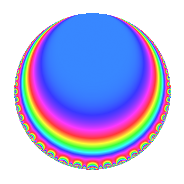Properties

 Label 1225.1.g.aLevel 1225 Weight 1 Character orbit 1225.g Analytic conductor 0.611 Analytic rank 0 Dimension 2 Projective image $$D_{2}$$ CM/RM disc. -7, -35, 5 Inner twists 8

Related objects

Newspace parameters

 Level: $$N$$ = $$1225 = 5^{2} \cdot 7^{2}$$ Weight: $$k$$ = $$1$$ Character orbit: $$[\chi]$$ = 1225.g (of order $$4$$ and degree $$2$$)

Newform invariants

 Self dual: No Analytic conductor: $$0.611354640475$$ Analytic rank: $$0$$ Dimension: $$2$$ Coefficient field: $$\Q(i)$$ Coefficient ring: $$\Z[a_1, \ldots, a_{4}]$$ Coefficient ring index: $$1$$ Projective image $$D_{2}$$ Projective field Galois closure of $$\Q(\sqrt{5}, \sqrt{-7})$$ Artin image size $$16$$ Artin image $OD_{16}$ Artin field Galois closure of 8.4.9191328125.1

$q$-expansion

The $$q$$-expansion and trace form are shown below.

 $$f(q)$$ $$=$$ $$q$$ $$-i q^{4}$$ $$+ i q^{9}$$ $$+O(q^{10})$$ $$q$$ $$-i q^{4}$$ $$+ i q^{9}$$ $$+ 2 q^{11}$$ $$- q^{16}$$ $$-2 i q^{29}$$ $$+ q^{36}$$ $$-2 i q^{44}$$ $$+ i q^{64}$$ $$-2 q^{71}$$ $$+ 2 i q^{79}$$ $$- q^{81}$$ $$+ 2 i q^{99}$$ $$+O(q^{100})$$ $$\operatorname{Tr}(f)(q)$$ $$=$$ $$2q$$ $$\mathstrut +\mathstrut O(q^{10})$$ $$2q$$ $$\mathstrut +\mathstrut 4q^{11}$$ $$\mathstrut -\mathstrut 2q^{16}$$ $$\mathstrut +\mathstrut 2q^{36}$$ $$\mathstrut -\mathstrut 4q^{71}$$ $$\mathstrut -\mathstrut 2q^{81}$$ $$\mathstrut +\mathstrut O(q^{100})$$

Character Values

We give the values of $$\chi$$ on generators for $$\left(\mathbb{Z}/1225\mathbb{Z}\right)^\times$$.

 $$n$$ $$101$$ $$1177$$ $$\chi(n)$$ $$1$$ $$i$$

Embeddings

For each embedding $$\iota_m$$ of the coefficient field, the values $$\iota_m(a_n)$$ are shown below.

For more information on an embedded modular form you can click on its label.

Label $$\iota_m(\nu)$$ $$a_{2}$$ $$a_{3}$$ $$a_{4}$$ $$a_{5}$$ $$a_{6}$$ $$a_{7}$$ $$a_{8}$$ $$a_{9}$$ $$a_{10}$$
393.1
 − 1.00000i 1.00000i
0 0 1.00000i 0 0 0 0 1.00000i 0
932.1 0 0 1.00000i 0 0 0 0 1.00000i 0
 $$n$$: e.g. 2-40 or 990-1000 Significant digits: Format: Complex embeddings Normalized embeddings Satake parameters Satake angles

Inner twists

Char. orbit Parity Mult. Self Twist Proved
1.a Even 1 trivial yes
5.b Even 1 RM by $$\Q(\sqrt{5})$$ yes
7.b Odd 1 CM by $$\Q(\sqrt{-7})$$ yes
35.c Odd 1 CM by $$\Q(\sqrt{-35})$$ yes
5.c Odd 2 yes
35.f Even 2 yes

Hecke kernels

This newform can be constructed as the kernel of the linear operator $$T_{2}$$ acting on $$S_{1}^{\mathrm{new}}(1225, [\chi])$$.